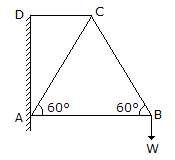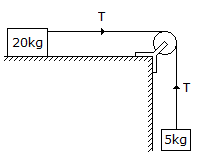Last updated: August 14, 2020

1. The polygon law of forces states that if a number of forces, acting simultaneously on a particle, be represented in magnitude and direction by the sides a polygon taken in order, then their resultant is represented in magnitude and direction by the closing side of the polygon, taken in opposite direction.
2. The maximum height of a projectile on a horizontal plane, is
3. The centre of gravity of a right circular solid cone is at a distance of __________ from its base, measured along the vertical axis.(where h = Height of a right circular solid cone.)
4. Can an irregular body have more than one centre of gravity?
5. If the masses of both the bodies, as shown in the below figure, are doubled, then the acceleration in the string will be6. One end of a helical spring is fixed while the other end carries the load W which moves with simple harmonic motion. The frequency of motion is given by(where δ = Deflection of the spring.)
7. Moment of inertia of a rectangular section having width (b) and depth (d) about an axis passing through its C.G. and parallel to the depth (d), is
8. The centre of oscillation and centre of suspension for a compound pendulum are interchangeable.
9. In actual machines, mechanical advantage is __________ velocity ratio.
10. In a framed structure, as shown in the below figure, the force in the member CD is tensile in nature.11. A rubber ball is dropped from a height of 2 m. If there is no loss of velocity after rebounding, the ball will rise to a height of
12. The centre of gravity of a hemisphere lies at a distance of 3r / 8 from its base measured along the vertical radius.
13. Moment of inertia of a circular section about its diameter (d) is
14. The radius of gyration is the distance where the whole mass (or area) of a body is assumed to be concentrated.
15. A smooth cylinder lying on a __________ is in neutral equilibrium.
16. The loss of kinetic energy during inelastic impact, is given by(where m1 = Mass of the first body, m2 = Mass of the second body, and u1 and u2 = Velocities of the first and second bodies respectively.)
17. In a wormed geared pulley block, if the number of teeth on the worm wheel is doubled, then its velocity ratio is also doubled.
18. If three coplaner forces acting on a point are in equilibrium, then each force is proportional to the sine, of the angle between the other two.
19. A block ot mass 20 kg lying on a rough horizontal plane is connected by a light string passing over a smooth pulley to another mass 5 kg, which can move freely in the Vertical direction, as shown in the below figure. The tension in the string will __________ with the increase in coefficient of friction.20. When the spring of a watch is wound, it will possess
Engineering Mechanics MCQ 6
You got {{userScore}} out of {{maxScore}} correct
{{title}}
{{image}}
{{content}}